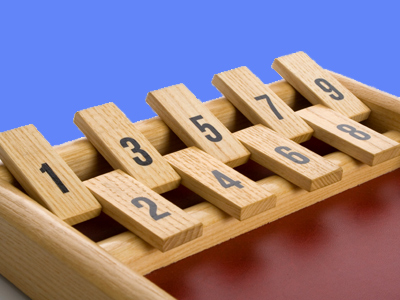Even numbers can all be divided by 2 - odd numbers cannot.

# More Odd or Even

This Math quiz is called 'More Odd or Even' and it has been written by teachers to help you if you are studying the subject at elementary school. Playing educational quizzes is a fun way to learn if you are in the 1st or 2nd grade - aged 6 to 8.

It costs only \$12.50 per month to play this quiz and over 3,500 others that help you with your school work. You can subscribe on the page at Join Us

Recognizing odd or even numbers is usually a highly developed skill after a year in elementary school. Children should be able to use odd and even when talking about the properties of number, as well as using this recognition to create and extend larger and more complex number sequences, including those using higher numbers over 100.

Try this quiz and see if you can recognize odd or even numbers.

1.
Even numbers can always be...
divided equally by 2
larger than odd numbers
smaller than odd numbers
divided equally by 3
Even numbers can be divided by two and give a whole number as an answer
2.
What is wrong with this sequence: 107, 109, 110, 111, 113, 115
Some numbers have a zero digit
The numbers all have 3 digits
It is too long
It contains an even number
The sequence is all odd numbers, apart from 110, which is even
3.
Which set of numbers is the odd one out?
16, 28, 36, 54
10, 30, 44, 68
13, 91, 103, 121
18, 74, 82, 104
The other sets are even, this set contains only odd numbers
4.
What are the next two numbers in this sequence: 33, 35, 37, 39
34, 36
36, 38
40, 41
41, 43
The sequence is made up of odd numbers
5.
If you add an even and an odd number together, the answer is always...
an even number
a number higher than 7
a number lower than 12
an odd number
For example, 5 + 4 = 9 or 15 + 10 = 25
6.
Which is the next number in this sequence: 15, 17, 19, ?
18
20
21
23
The numbers are all odd, and are going up in twos
7.
Which number isn't even?
27
36
82
90
Even numbers always end with 2, 4, 6, 8 or 0
8.
Which set contains an odd number?
26, 88, 104, 268
54, 67, 108, 234
22, 64, 120, 238
44, 50, 82, 156
67 ends with a '7' and is odd
9.
Which statement is true?
Even numbers are all larger than 10
Odd numbers are sometimes in the 2 times table
Even numbers always end with 2, 4, 6, 8 or 0
Odd numbers always end with 3
Odd numbers end with 1, 3, 5, 7 or 9
10.
When you add two even numbers together, the result is always...
an odd number
an even number
a number higher than 10
a number less than 50